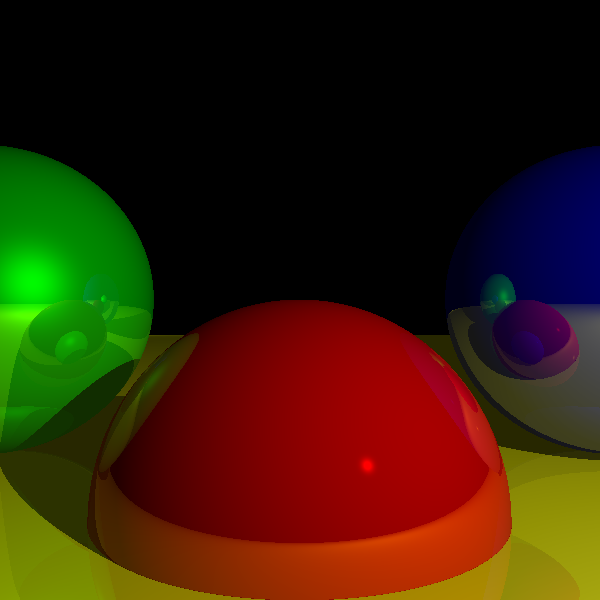# Tiny Raytracer

I love raytracers. Their combination of a simple algorithm and stunning results are hard to beat.

I’ve written several raytracers over the years, but this time I decided to write the smallest possible one I could write. My current version is 912 bytes of Javascript, and I’m sure it’s still possible to shave off a few more bytes. Note that the size includes a simple scene definition.

Here’s the full source code:

`var G=600,S=[G,[0,-G,0],9,9,0,G,2,1,[0,0,3],9,0,0,G,3,1,[-2,1,4],0,9,0,9,4,1,[2,1,4],0,0,9,G,5],a=2,L=[8,[2,2,0]],M=Math,Q=M.sqrt,X=M.max,o=0,C=document.getElementById("c"),Z=C.getContext("2d"),m=Z.getImageData(0,0,G,G),W=m.data,d=(A,B)=>A*B+A*B+A*B,A=(A,B,k)=>[A-B*k,A-B*k,A-B*k],I=(O,D,m,M)=>{t=G;for(c=i=0;r=S[i++];i+=6)if(j=A(O,S[i],1),a=2*d(D,D),b=-2*d(j,D),k=Q(b*b-2*a*(d(j,j)-r*r)))for(e=2;e--;k=-k)f=(b-k)/a,m<f&&f<M&&f<t&&(c=i,t=f);return c},T=(O,D,m,r)=>{if(s=I(O,D,m,G)){N=A(P=A(O,D,-t),S[s],1);n=d(N,N);i=a;for(l=0;e=L[l++];)p=d(N,v=A(L[l++],P,1)),i+=e*!I(P,v,1/G,1)*(X(0,p/Q(d(v,v)*n))+X(0,M.pow(d(R=A(v,N,2*p/n),D)/Q(d(R,R)*d(D,D)),S[s+4])));O=S[s+H]*i*2.8;m=S[s+5]/9;return r--?(T(P,A(D,N,2*d(N,D)/n),1/G,r)|0)*m+O*(1-m):O}};C.width=C.height=G;for(y=h=G/2;y-->-h;)for(x=-h;x++<h;){for(H=0;4>++H;)W[o++]=T([0,1,0],[x/G,y/G,1],1,2);W[o++]=G}Z.putImageData(m,0,0);`

And here’s the output:The code above was generated by Google’s Closure Compiler, plus some manual tweaks. The original, fully commented source code can be found here. Note that the goal here was to make the source code as small as possible, not clarity; so even the original code before minification is a horrible mess. This doesn’t do justice to the elegance and simplicity of proper raytracer code; I’m writing a book to right this wrong.

It should run in any reasonable browser that supports the `<canvas>` tag and has a good Javascript interpreter. Here’s a live demo.

You might also enjoy my book Computer Graphics From Scratch.

Found this interesting?